# YEAR 2 Length Height Measure length cm Measure

• Slides: 46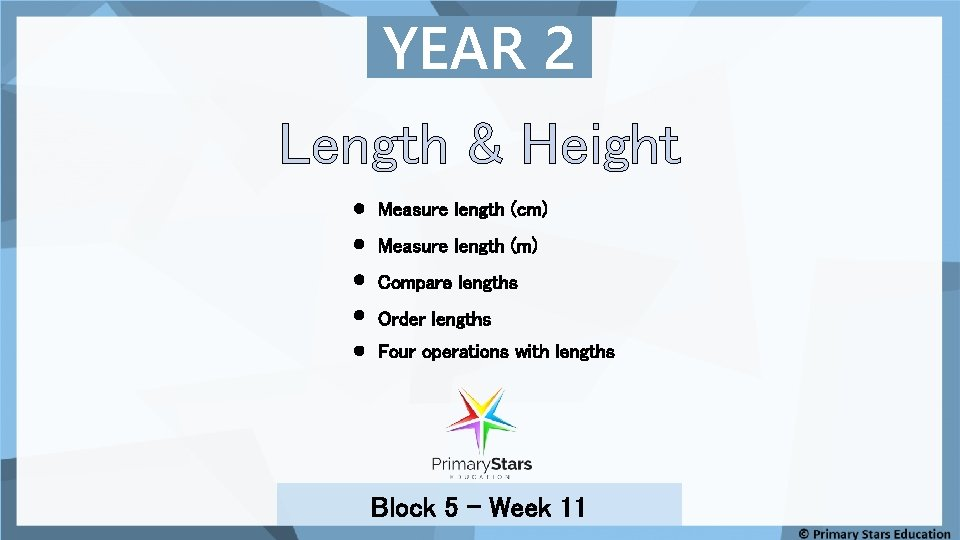YEAR 2 Length & Height Measure length (cm) Measure length (m) Compare lengths Order lengths Four operations with lengths Block 5 – Week 11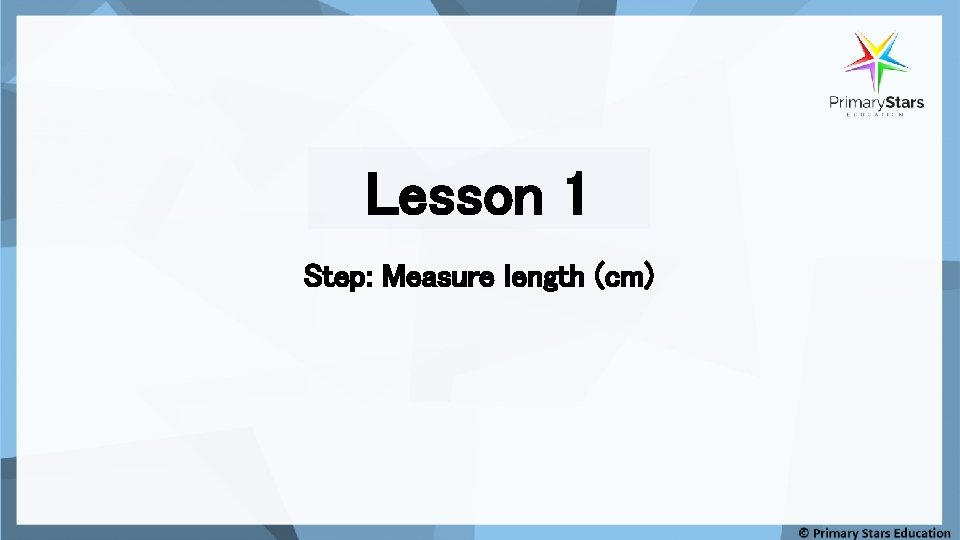Lesson 1 Step: Measure length (cm)Fluency Recap: Remember to line up the item at 0 when measuring. Each mark is 1 cm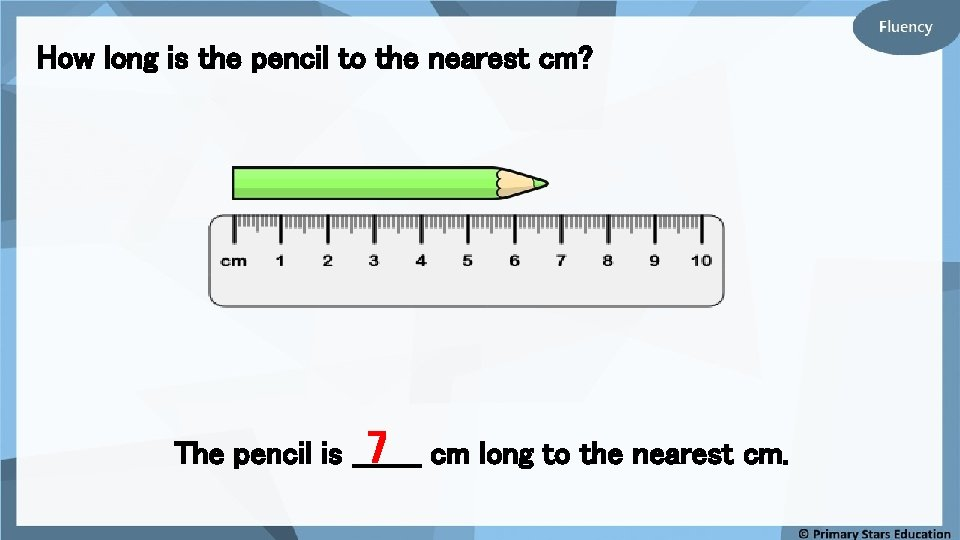How long is the pencil to the nearest cm? 7 cm long to the nearest cm. The pencil is _______How long is the pencil to the nearest cm? 5 cm long to the nearest cm. The pencil is _______How long is the pencil to the nearest cm? 9 cm long to the nearest cm. The pencil is _______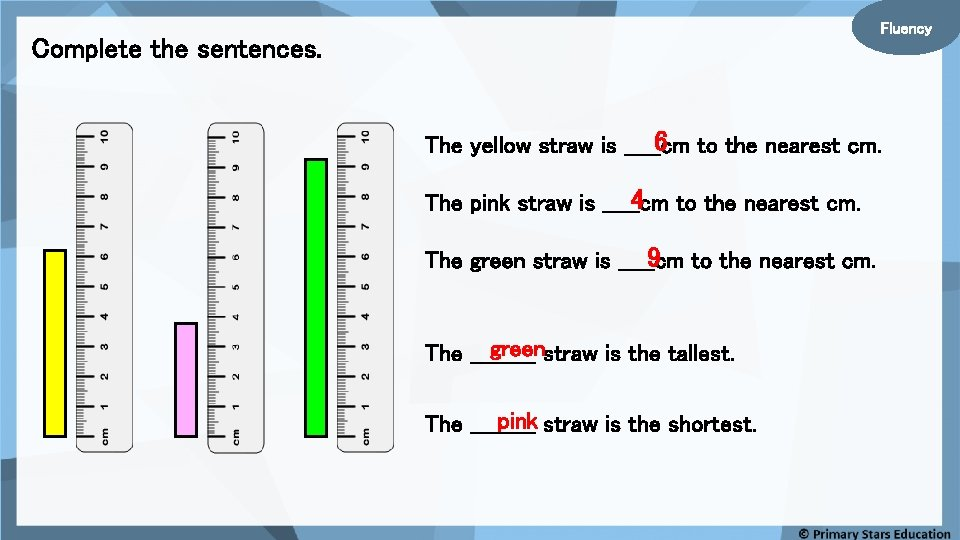Fluency Complete the sentences. 6 to the nearest cm. The yellow straw is _____cm 4 to the nearest cm. The pink straw is _____cm 9 to the nearest cm. The green straw is _____cm greenstraw is the tallest. The _____ pink straw is the shortest. The _____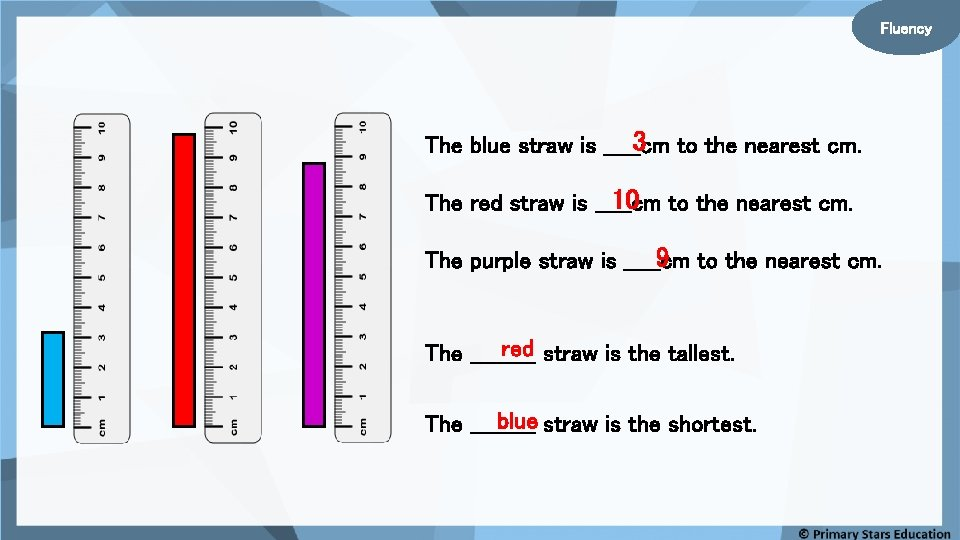Fluency 3 to the nearest cm. The blue straw is _____cm 10 to the nearest cm. The red straw is _____cm 9 to the nearest cm. The purple straw is _____cm red straw is the tallest. The _____ blue straw is the shortest. The _____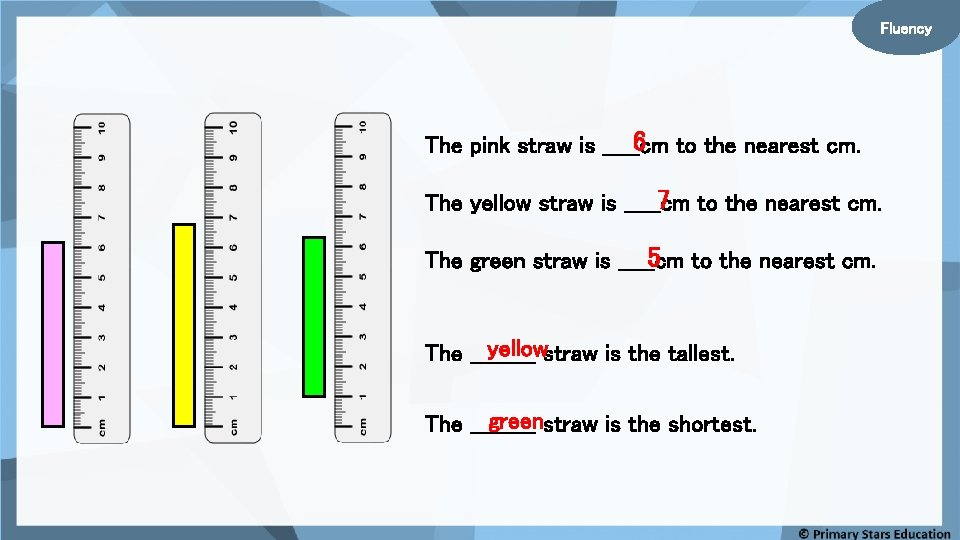Fluency 6 to the nearest cm. The pink straw is _____cm 7 to the nearest cm. The yellow straw is _____cm 5 to the nearest cm. The green straw is _____cm yellowstraw is the tallest. The _____ greenstraw is the shortest. The _____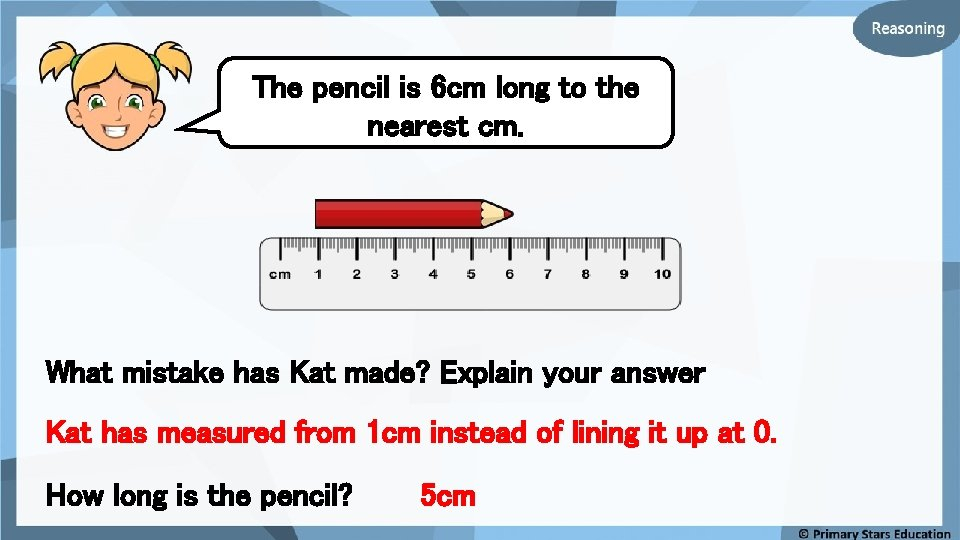The pencil is 6 cm long to the nearest cm. What mistake has Kat made? Explain your answer Kat has measured from 1 cm instead of lining it up at 0. How long is the pencil? 5 cm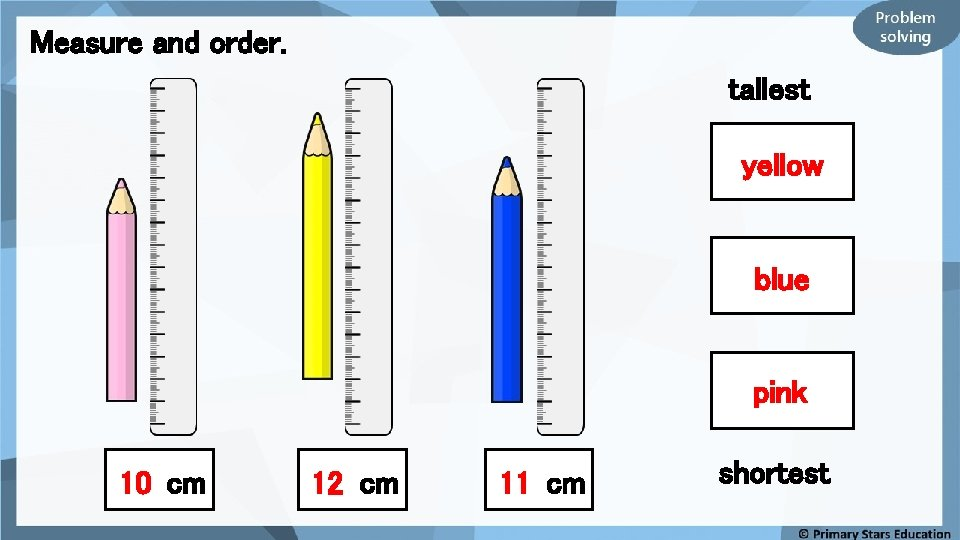Measure and order. tallest yellow blue pink 10 cm 12 cm 11 cm shortest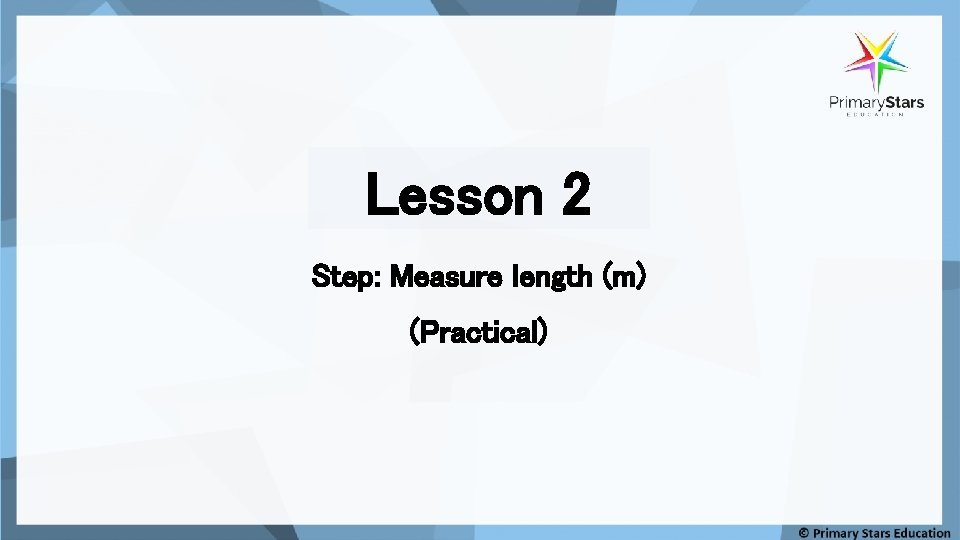Lesson 2 Step: Measure length (m) (Practical)Fluency If we measured the size of the classroom, would we use a ruler? What could we use and why would this be better? Metre stick or tape measureOutdoor activity: Fluency Measure how big you think one metre is with a piece of chalk. How close was your estimate?Fluency Using a metre stick measure: Chair Can you name an item that is bigger? . . smaller?Fluency Using a metre stick measure: Table Can you name an item that is bigger? . . smaller?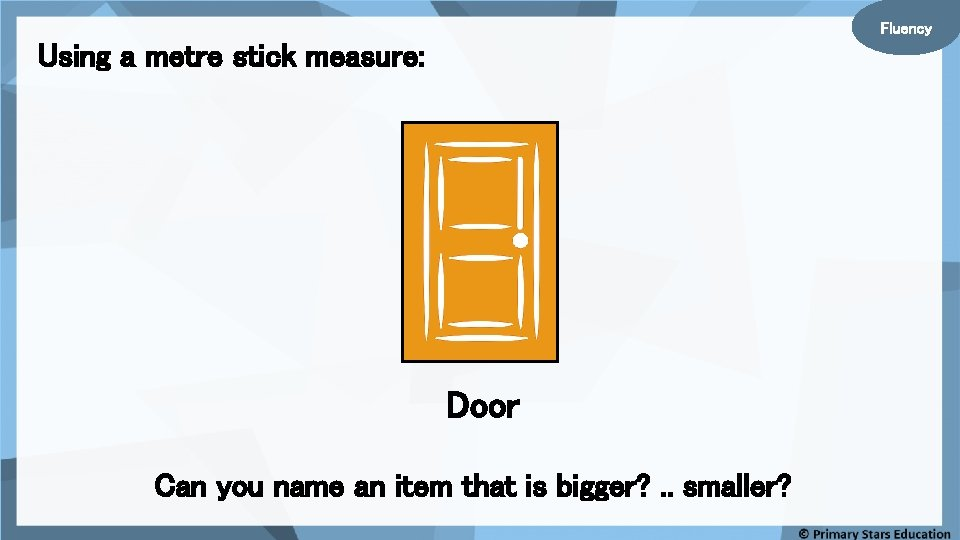Fluency Using a metre stick measure: Door Can you name an item that is bigger? . . smaller?Fluency What items could be measured using? . . Ruler Metre stick Why would you use one over the other?Fluency Sort the objects in the table below. Less than 1 m Bigger than 1 mSue is measuring around the stone which is more than 1 m. What could I use to measure the stone as I am struggling with a metre stick? Sue could use a tape measure to measure around the stone.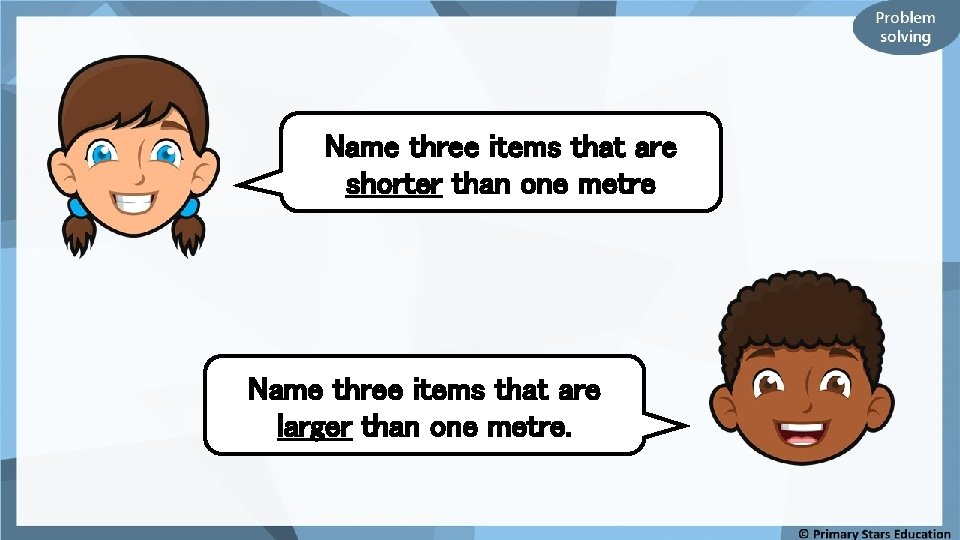Name three items that are shorter than one metre Name three items that are larger than one metre.Lesson 3 Step: Compare lengths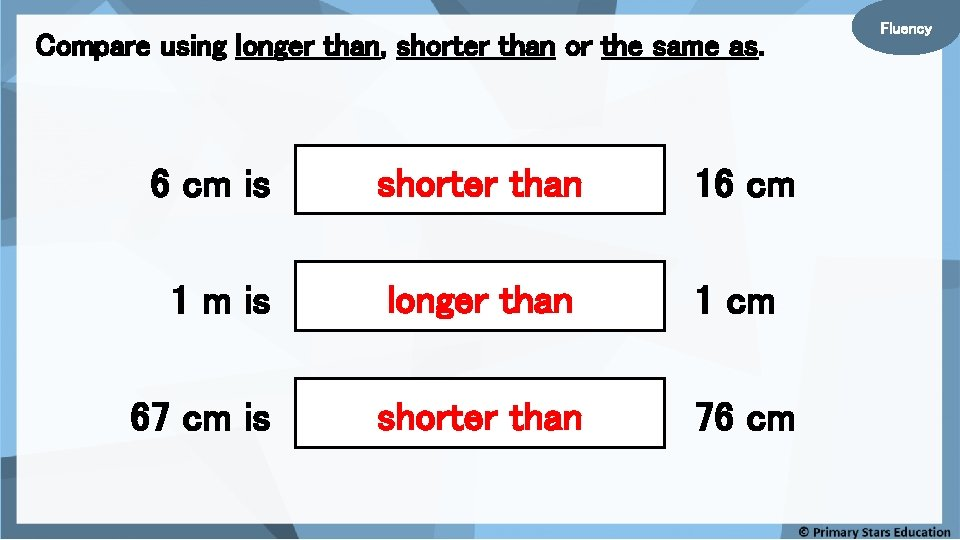Compare using longer than, shorter than or the same as. 6 cm is shorter than 16 cm 1 m is longer than 1 cm 67 cm is shorter than 76 cm Fluency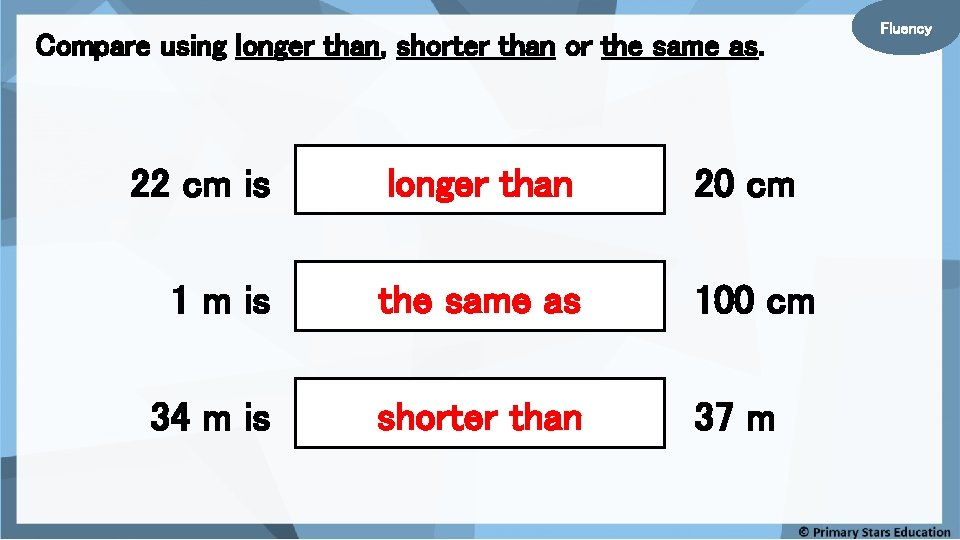Compare using longer than, shorter than or the same as. 22 cm is longer than 20 cm 1 m is the same as 100 cm 34 m is shorter than 37 m Fluency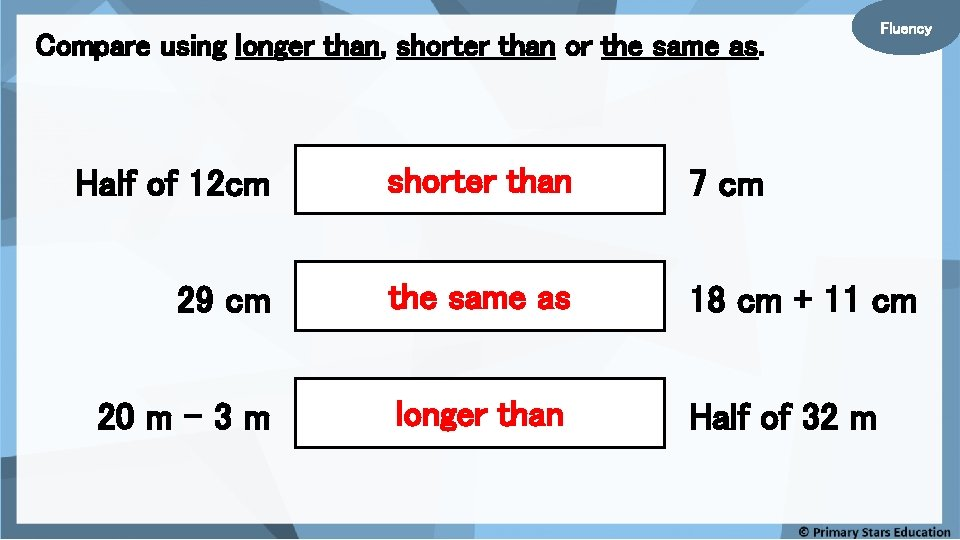Compare using longer than, shorter than or the same as. Fluency Half of 12 cm shorter than 7 cm 29 cm the same as 18 cm + 11 cm 20 m – 3 m longer than Half of 32 mFluency Compare using > , < or = 13 cm is < 31 cm 96 m is > 89 m 47 cm is < 50 cm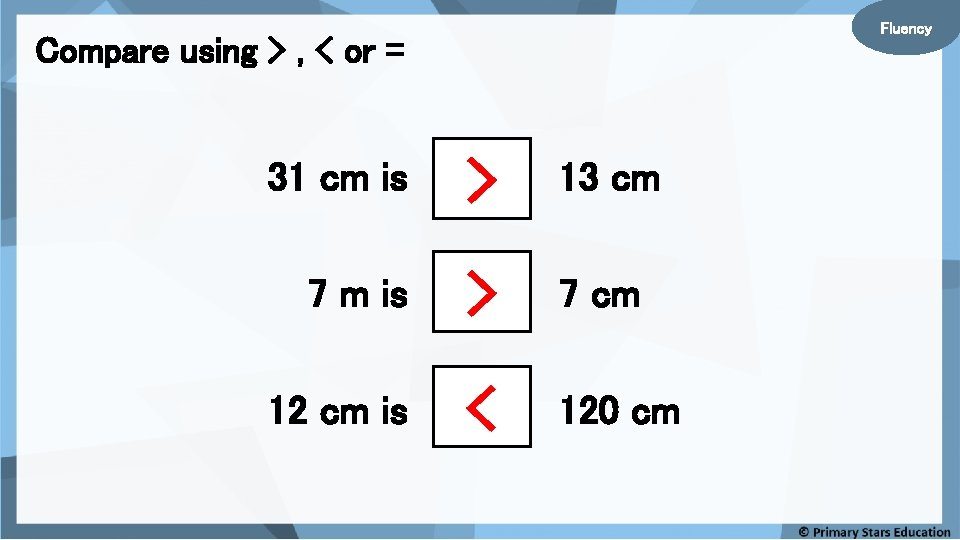Fluency Compare using > , < or = 31 cm is > 13 cm 7 m is > 7 cm 12 cm is < 120 cm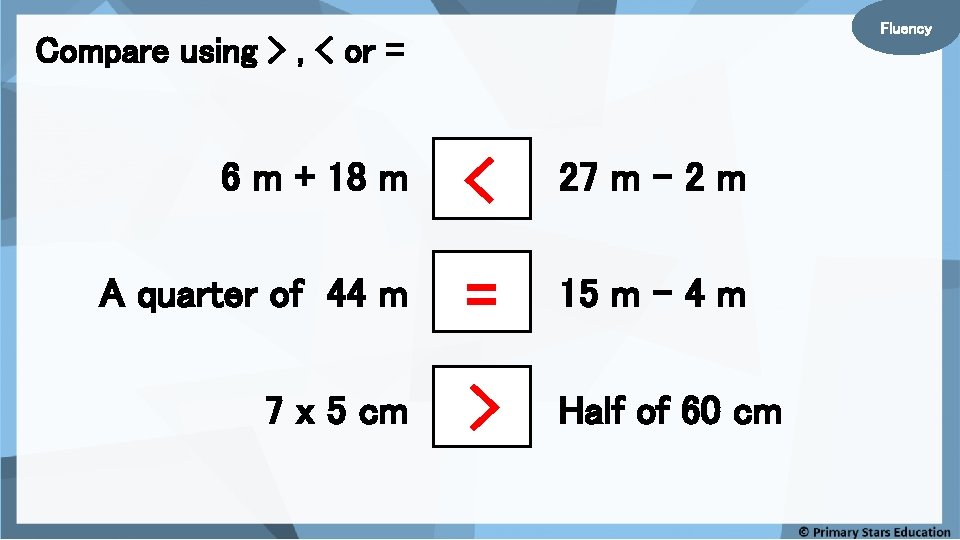Fluency Compare using > , < or = 6 m + 18 m < 27 m – 2 m A quarter of 44 m = 15 m – 4 m 7 x 5 cm > Half of 60 cm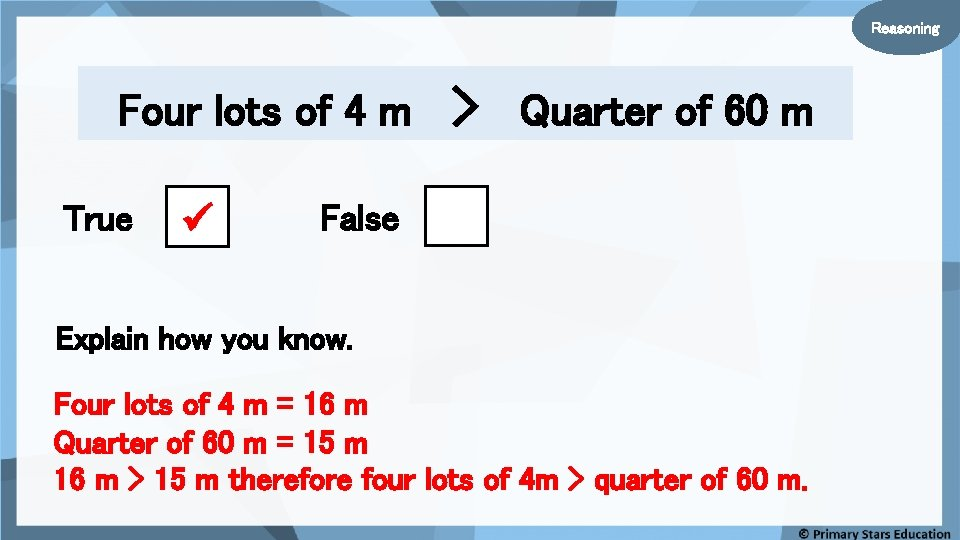Reasoning Four lots of 4 m True > Quarter of 60 m False Explain how you know. Four lots of 4 m = 16 m Quarter of 60 m = 15 m 16 m > 15 m therefore four lots of 4 m > quarter of 60 m.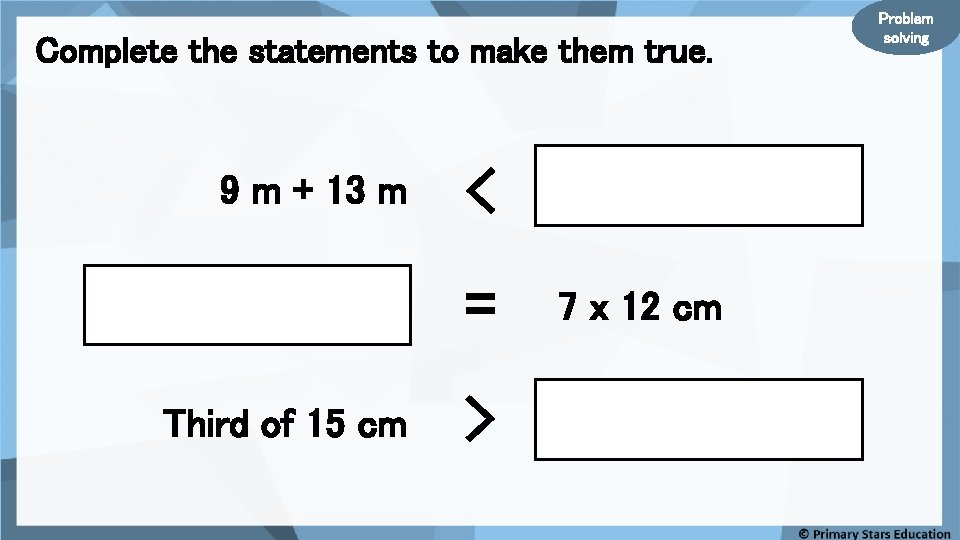Complete the statements to make them true. 9 m + 13 m < = Third of 15 cm > 7 x 12 cm Problem solvingLesson 4 Step: Order lengths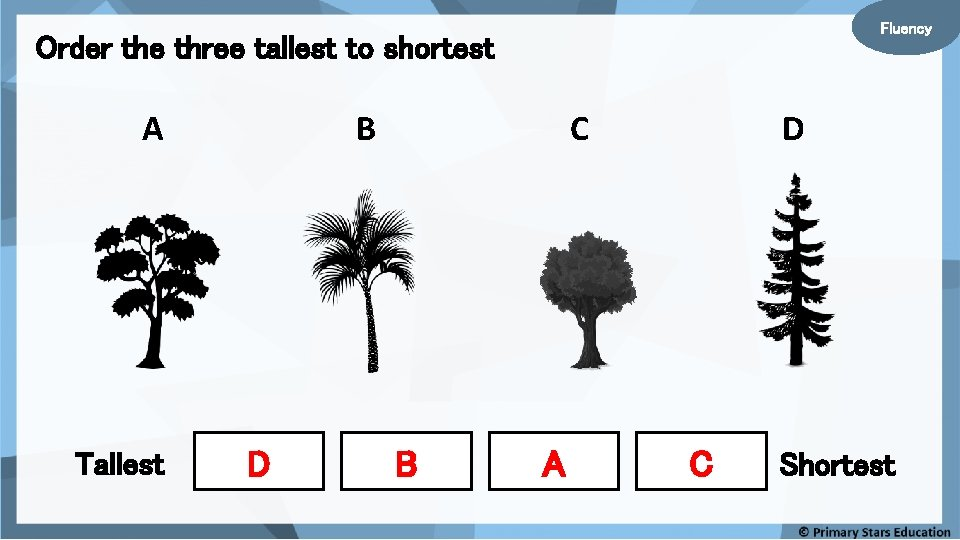Fluency Order the three tallest to shortest A Tallest B D C B A D C Shortest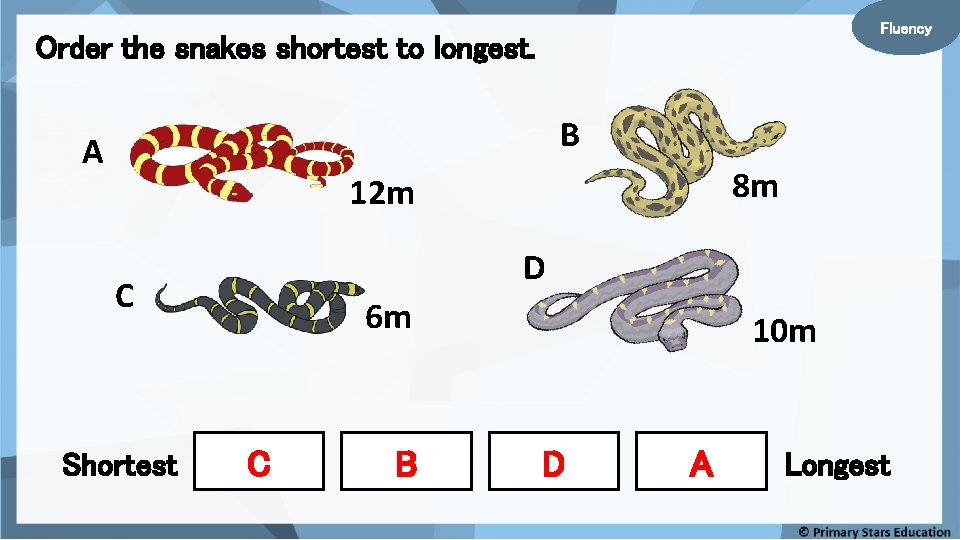Fluency Order the snakes shortest to longest. B A 8 m 12 m D C Shortest 6 m C B 10 m D A Longest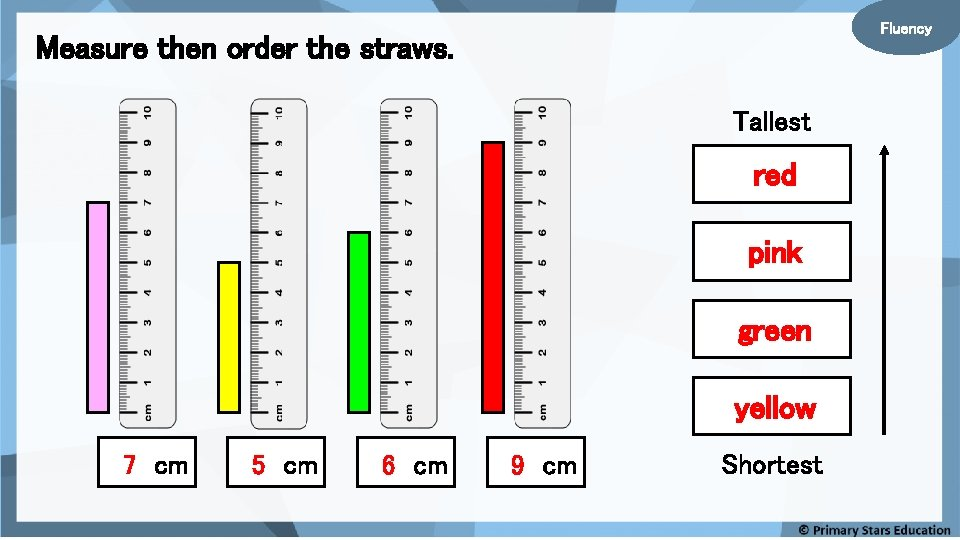Fluency Measure then order the straws. Tallest red pink green yellow 7 cm 5 cm 6 cm 9 cm Shortest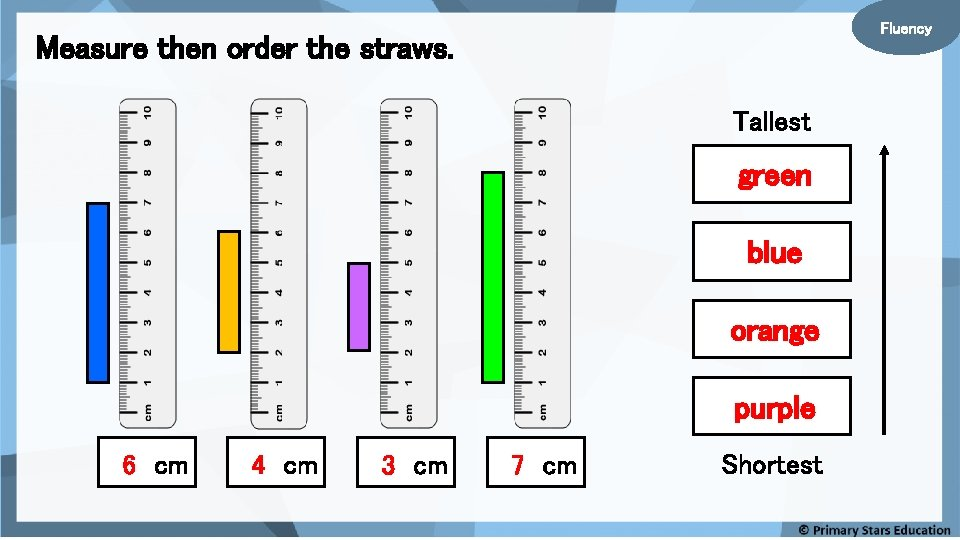Fluency Measure then order the straws. Tallest green blue orange purple 6 cm 4 cm 3 cm 7 cm ShortestFluency Order the following lengths shortest to longest. 21 m 19 m 9 m 11 m Shortest 9 m 11 m 19 m 21 m LongestFluency Order the following lengths shortest to longest. 1 m m c 5 6 m c 6 5 m c 1 1 Longest 1 m 65 cm 56 cm 11 cm ShortestThe length that should go in the box is 18 m. Shortest 6 x 3 m ? Is Sue correct? Explain your answer. No, Sue is not correct. The length would go in the box is 19 m. Longest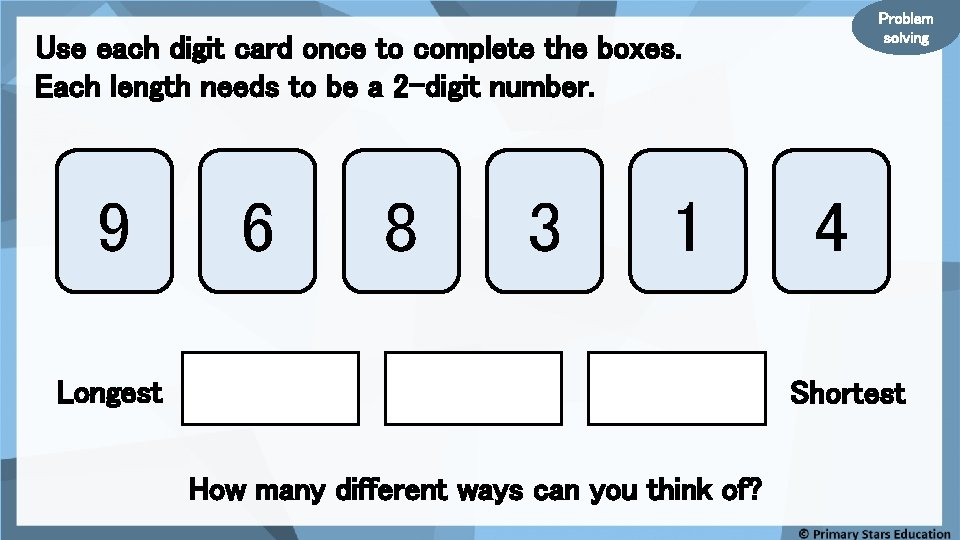Problem solving Use each digit card once to complete the boxes. Each length needs to be a 2 -digit number. 9 6 8 3 1 Longest 4 Shortest How many different ways can you think of?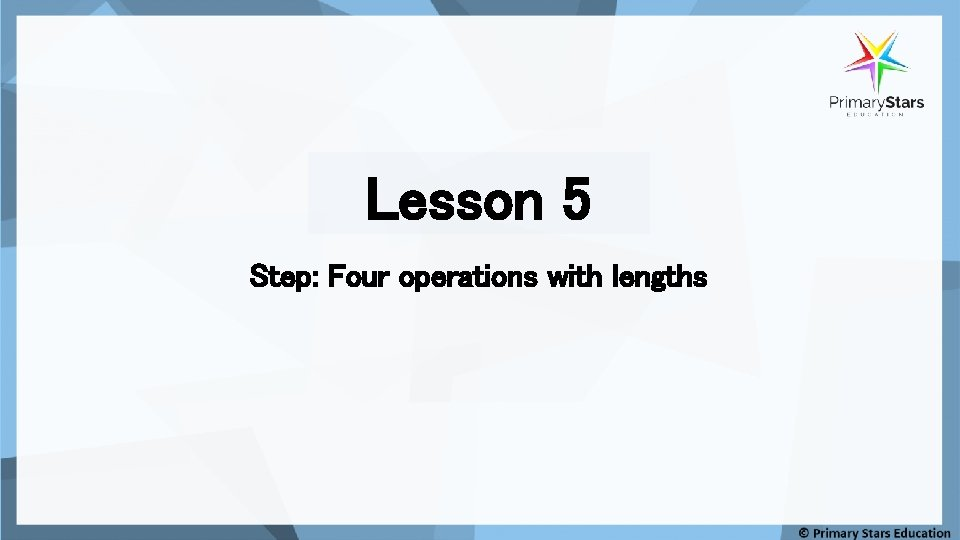Lesson 5 Step: Four operations with lengths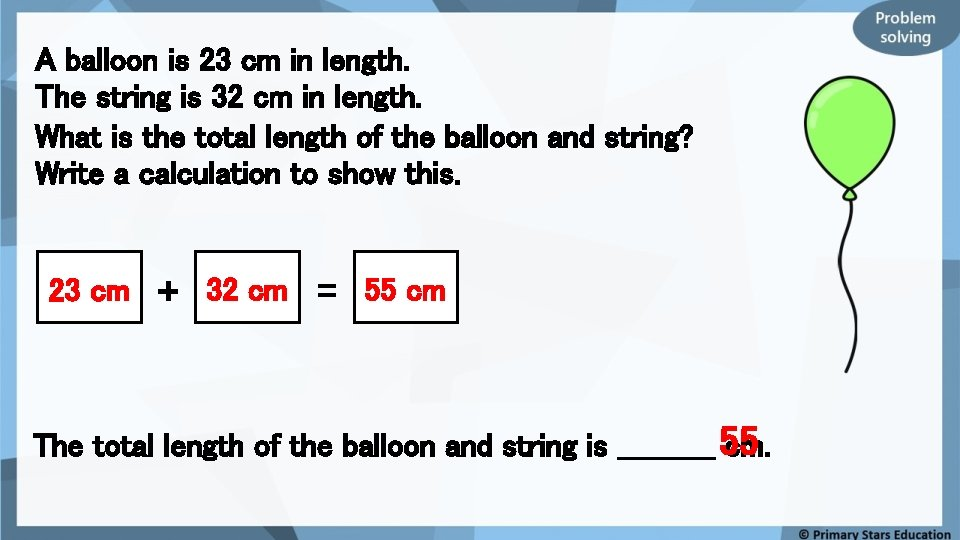A balloon is 23 cm in length. The string is 32 cm in length. What is the total length of the balloon and string? Write a calculation to show this. 23 cm + 32 cm = 55 cm The total length of the balloon and string is _____ 55 cm.String A measures 17 cm long. String B measures 16 cm long. What is the combined length of both strings? Write a calculation to show this. 17 cm + 16 cm = 33 cm 33 cm. The total length of string is _____ Problem solving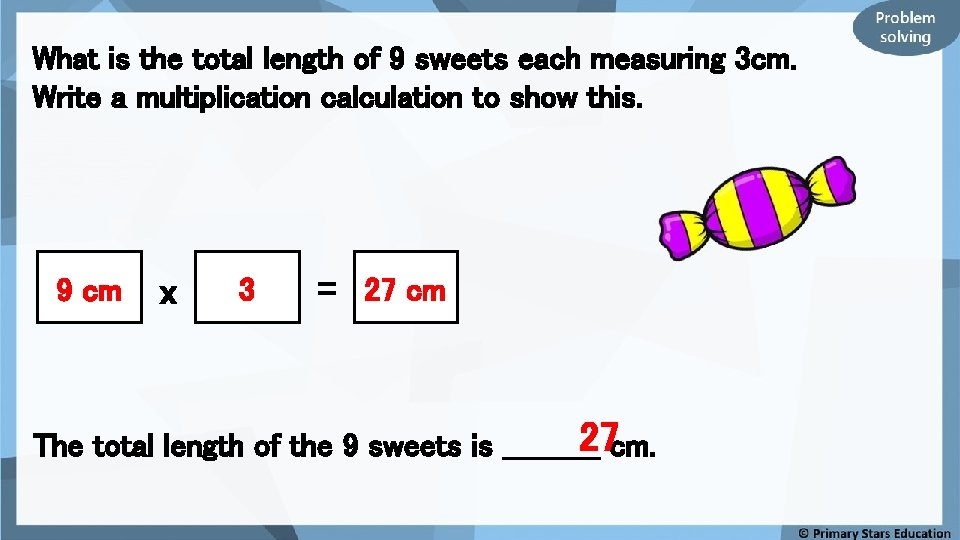What is the total length of 9 sweets each measuring 3 cm. Write a multiplication calculation to show this. 9 cm x 3 = 27 cm 27 cm. The total length of the 9 sweets is _____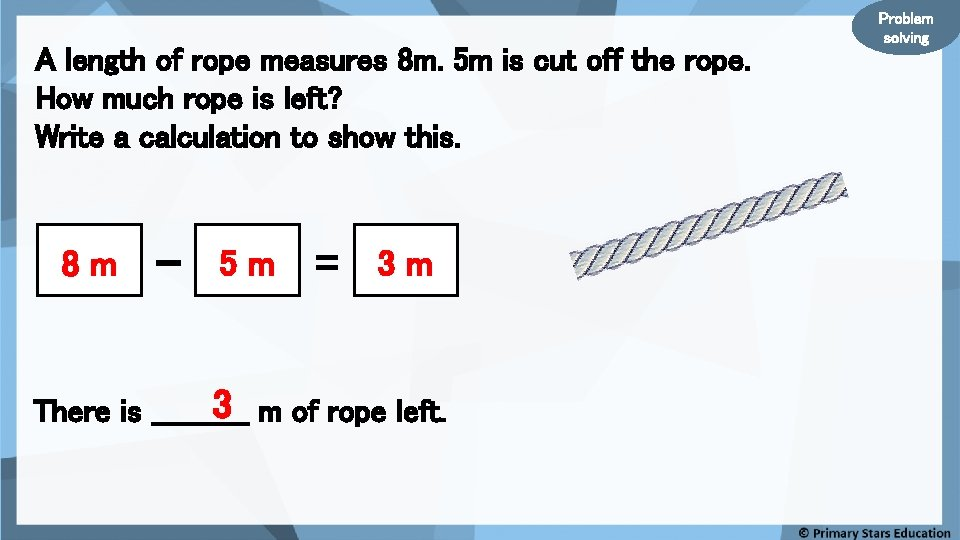A length of rope measures 8 m. 5 m is cut off the rope. How much rope is left? Write a calculation to show this. 8 m - 5 m = 3 m 3 m of rope left. There is _____ Problem solving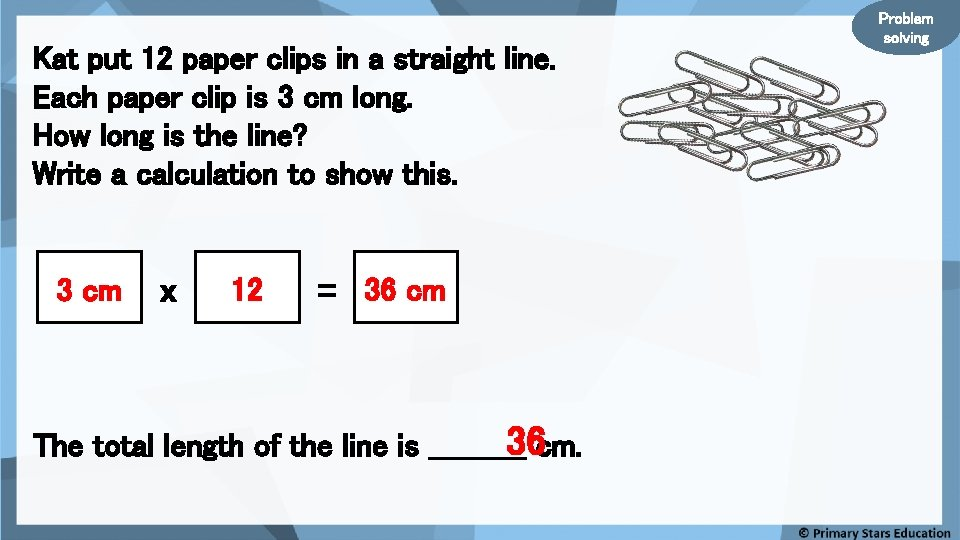Kat put 12 paper clips in a straight line. Each paper clip is 3 cm long. How long is the line? Write a calculation to show this. 3 cm x 12 = 36 cm 36 cm. The total length of the line is _____ Problem solvingRibbon A is 13 cm. Ribbon B is 10 cm. Ribbon C is 4 cm longer than Ribbon A. What is the total length of the three ribbons combined? Write a calculation to show this. 13 cm + 10 cm + 17 cm = 40 cm. Can you represent this on a bar model? . . Part-whole model? Problem solving